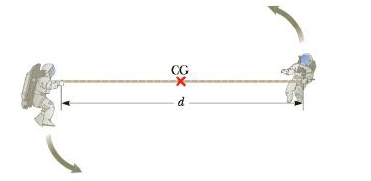Chapter 8, Problem 80AP

Chapter
Section
Textbook Problem

Two astronauts (Fig. P8.80), each haring a mass of 75.0 kg, are connected by a 10.0-m rope of negligible mass. They are isolated in space, moving in circles around the point halfway between them at a speed of 5.00 m/s. Treating the astronauts as particles, calculate (a) the magnitude of the angular momentum and (b) the rotational energy of the system. By pulling on the rope, the astronauts shorten the distance between them to 5.00 m. (c) What is the new angular momentum of the system? (d) What are their new’ speeds? (e) What is the new rotational energy of the system? (f) How much work is done by the astronauts in shortening the rope?Figure P8.80 Problems 80 and 81

(a)

To determine
The angular momentum of the astronauts.

Explanation

Given Info:

The mass of the astronauts is 75kg , the speed of the astronauts is 5.00ms1 , the distance between them is 10.0m .

The formula to calculate angular momentum is given by

Li=mviri

• Li is the initial angular momentum,
• m is the mass of astronauts
• vi is initial the speed of the astronauts
• ri is the initial distance between the astronaut from the centre of mass.

Substitute 75kg for m, 5.00ms1 for vi and 10.0m for ri in the above expression to calculate Li

(b)

To determine
The rotational energy of the system.

(c)

To determine
The angular momentum of the system for the shortened distance.

(d)

To determine
speed of the astronauts

(e)

To determine
The new rotational energy of the system.

(f)

To determine
The work done by the astronauts.

Still sussing out bartleby?

Check out a sample textbook solution.

See a sample solution

The Solution to Your Study Problems

Bartleby provides explanations to thousands of textbook problems written by our experts, many with advanced degrees!

Get Started